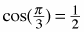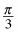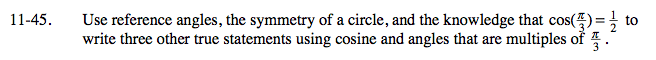### Home > A2C > Chapter Ch11 > Lesson 11.2.1 > Problem11-45

11-45.

Use reference angles, the symmetry of a circle, and the knowledge thatto write three other true statements using cosine and angles that are multiples of. Homework Help ✎$\text{cos}\left(\frac{2\pi}{3}\right) = -\frac{1}{2}$

cos(π) = −1

$\text{cos}\left(-\frac{\pi}{3}\right) = \frac{1}{2}$

Now come up with your own examples.